# ISEE Upper Level Quantitative : How to find the length of the diagonal of a square

## Example Questions

### Example Question #1 : How To Find The Length Of The Diagonal Of A Square

Which is the greater quantity?

(a) The length of a diagonal of a square with sidelength 10 inches

(b) The hypotenuse of an isosceles right triangle with legs 10 inches each

It is impossible to tell which is greater from the information given.

(b) is greater.

(a) and (b) are equal.

(a) is greater.

(a) and (b) are equal.

Explanation:

A diagonal of a square cuts the square into two isosceles right triangles, of which the diagonal is the common hypotenuse. Therefore, each figure is the hypotenuse of an isosceles right triangle with legs 10 inches, making them equal in length.

### Example Question #1 : How To Find The Length Of The Diagonal Of A Square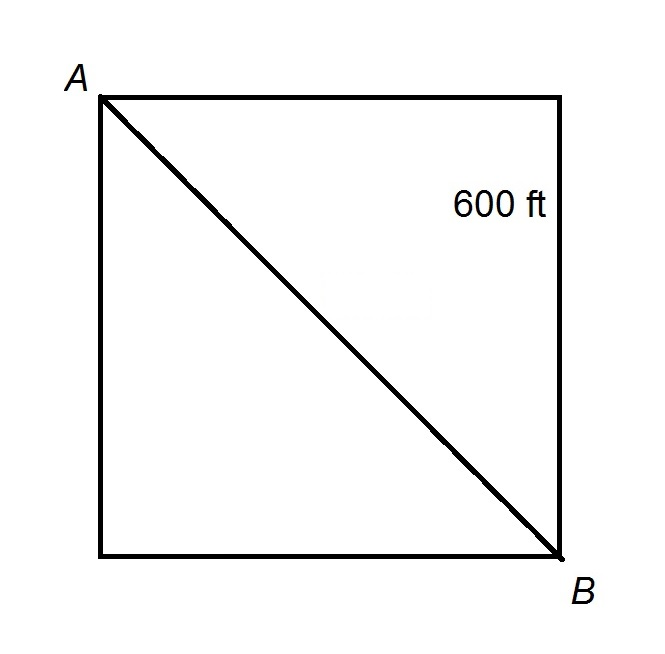The track at Peter Stuyvesant High School is a perfect square, as seen above, with sides of length 600 feet and a diagonal connecting two of the corners.

Les begins at Point A, takes the diagonal path directly to Point B, then runs counterclockwise around the square track twice. He then takes the diagonal from Point B back to Point A. Which of the following is closest to the distance he runs?

A hint: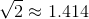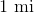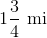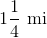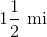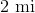Explanation:

The diagonal of a square has length, or about 1.414, times the length of a side, which here is 600 feet; this makes the diagonal path about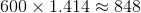feet long.

Les runs around the square track twice, meaning that he runs the length of one side eight times; he also runs the length of the diagonal twice, This is a total of about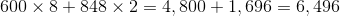feet.

Divide by 5,280 to convert to miles: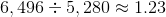Of the given responses,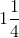miles comes closest to the correct distance.

### Example Question #3 : How To Find The Length Of The Diagonal Of A Square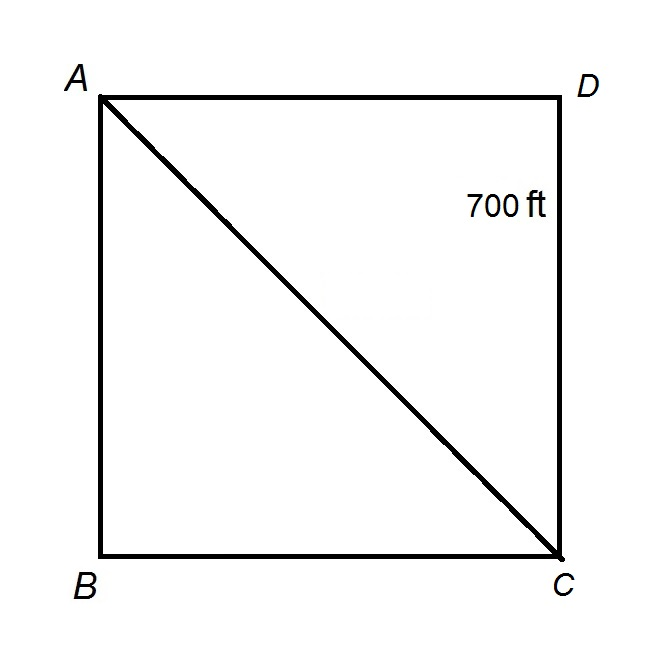The track at Franklin Pierce High School is a perfect square, as seen above, with sides of length 700 feet and a diagonal path connecting Points A and C.

Ellen wants to run three miles. Her plan is to begin at Point A, run along the diagonal path, run clockwise around the square track once, run along the diagonal path, run clockwise around the square track once, then repeat this pattern until she has run three miles. Where will she be when she is done?

A hint:On the square path between Point C and Point D

On the square path between Point D and Point A

On the square path between Point A and Point B

On the square path between Point B and Point C

On the diagonal path between Point A and Point C

On the diagonal path between Point A and Point C

Explanation:

The diagonal of a square has length, or about 1.414, times the length of a side, which here is 700 feet; this makes the diagonal path about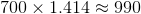feet long.

We will call one complete circuit one running of the diagonal, which is 990 feet long, and one running around the square; the completion of one complete circuit amounts to running a distance of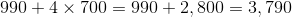feet.

Ellen seeks to run three miles, or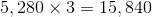feet, which, divided by 3,790 feet, is about: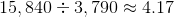,

or four complete circuits and 0.17 of a fifth.

After four complete circuits, Ellen is backat Point A. She has yet to run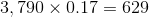feet.

She will now run along the diagonal from Point A to Point C, but since the diagonal has length 990 feet, which is greater than 629 feet, she will finish running three miles when she is on this diagonal path.

### Example Question #4 : How To Find The Length Of The Diagonal Of A Square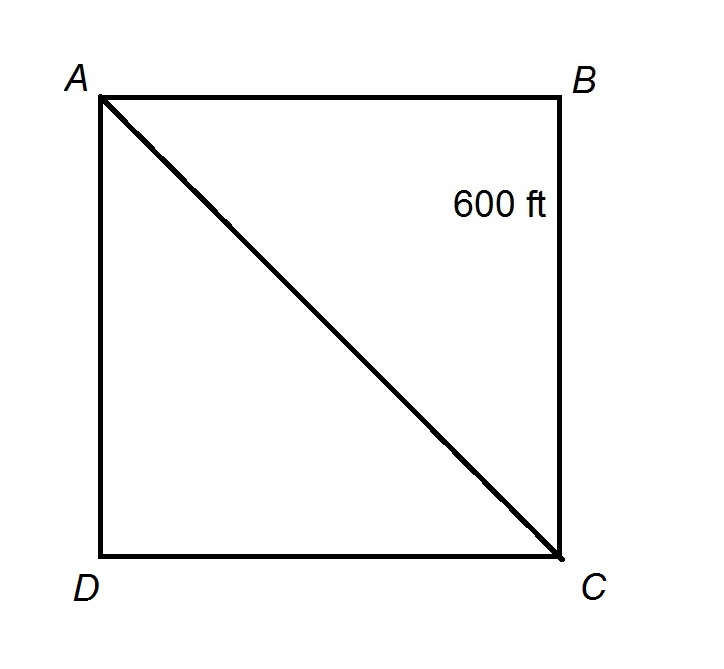The track at Grant High School is a perfect square, as seen above, with sides of length 600 feet and a diagonal path connecting two of the corners.

Kenny begins at Point A, runs the path to Point C, and proceeds to run counterclockwise around the square track one complete time. He then runs again along the diagonal path from Point C to Point A.

Which is the greater quantity?

(a) The length of Kenny's run

(b) One mile

A hint:(a) and (b) are equal

It is impossible to tell which is greater from the information given

(b) is greater

(a) is greater

(b) is greater

Explanation:

The diagonal of a square has length, or about 1.414, times the length of a side, which here is 600 feet; this makes the diagonal path aboutfeet long.

Kenny runs along this path twice, and he runs along the entire perimeter of the square path, so his run is about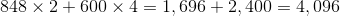feet. Since one mile is equal to 5,280 feet, the greater quantity is (b).